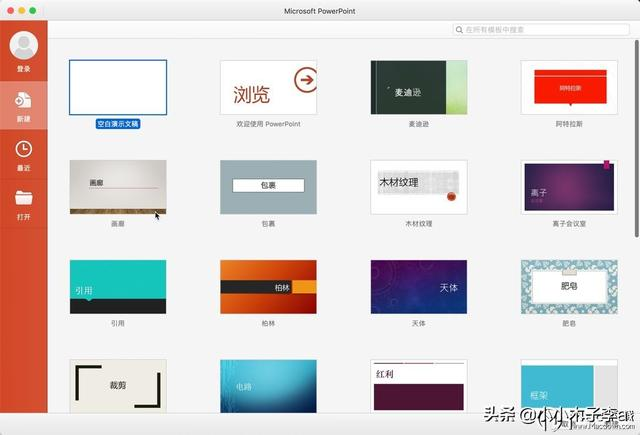PowerPoint for Mac 中的键盘快捷方式介绍给大家，使用PowerPoint for Mac可以创建新颖别致的演示文稿通过漂亮的设计、丰富的动画、电影动作、3D 模型和图标传达你的想法。利用智能技术的帮助，通过几次单击即可生动呈现演示文稿。PowerPoint for Mac 中的键盘快捷方式

Delete

FUNCTION + Delete

COMMAND + X

COMMAND + C

COMMAND + V

COMMAND + CONTROL + V

COMMAND + SHIFT + >

COMMAND + SHIFT + <

COMMAND + B

COMMAND + U

COMMAND + I

COMMAND + E

COMMAND + J

COMMAND + L

COMMAND + R

COMMAND + Y

COMMAND + Z

COMMAND + T

COMMAND + OPTION + M

OPTION + 向左键

OPTION + 向右键

COMMAND + 向右键

COMMAND + 向左键

OPTION + 向上键

OPTION + 向下键

COMMAND + 向上键/向下键

Tab

SHIFT + TAB

COMMAND + A

COMMAND + OPTION + G

COMMAND + OPTION + SHIFT + G

COMMAND + OPTION + J

OPTION + 向右键

OPTION + 向左键

COMMAND + SHIFT + 1

COMMAND + D

SHIFT + 箭头键

COMMAND + N

COMMAND + SHIFT + P

COMMAND + SHIFT + N

COMMAND + 减号

COMMAND +（加号）

COMMAND + SHIFT + D

COMMAND + O

COMMAND + W

COMMAND + P

COMMAND + S

COMMAND + SHIFT + S

COMMAND + Q

COMMAND + F

COMMAND + K

Esc

COMMAND + Z

COMMAND + Y

COMMAND + ~

COMMAND + SHIFT+ O

COMMAND + 1

COMMAND + 2

COMMAND + 3

COMMAND + 4

COMMAND + SHIFT + RETURN

COMMAND + CONTROL + F

OPTION + RETURN

COMMAND + OPTION + CONTROL + G

COMMAND + OPTION + 2 或 SHIFT + 单击“幻灯片浏览视图” “幻灯片浏览视图”按钮

COMMAND + OPTION + 1 或 SHIFT + 单击“普通视图” 普通视图按钮

COMMAND + OPTION + 3

SHIFT + 向右键

SHIFT + 向左键

SHIFT + 向上键

SHIFT + 向下键

COMMAND + SHIFT + 向左键

COMMAND + SHIFT + 向右键

SHIFT + OPTION + 向下键

SHIFT + OPTION + 向上键

N、PAGE DOWN、向右键、向下键或空格键（或单击鼠标按键）

P、Page Up、向左键、向上键或 DELETE

B

SHIFT + B

W

SHIFT + W

COMMAND + SHIFT + RETURN

COMMAND + RETURN

ESC、 COMMAND + 句点或连字符

E

SHIFT + E

H

COMMAND + I

COMMAND + U

COMMAND + P

COMMAND + A

CONTROL + H

COMMAND + OPTION + CONTROL + R

COMMAND + OPTION + CONTROL + L

OPTION + RETURN

TAB

SHIFT + TAB

RETURN

COMMAND + W

RETURN

Esc

COMMAND + H

COMMAND + M

COMMAND + CONTROL + Z